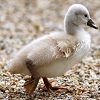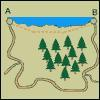#### You may also like### Walk and Ride

How far have these students walked by the time the teacher's car reaches them after their bus broke down?### Rolling Around

A circle rolls around the outside edge of a square so that its circumference always touches the edge of the square. Can you describe the locus of the centre of the circle?### N Is a Number

N people visit their friends staying N kilometres along the coast. Some walk along the cliff path at N km an hour, the rest go by car. How long is the road?

# Defined Distances

##### Age 11 to 14 Short Challenge Level:

Mark marked out the points $J$, $K$, $L$ and $M$ on a straight line, so that

$JK=13$cm
$KL=11$cm
$LM=14$cm
$MJ=12$cm

What is the distance, in cm, between the two points furthest apart?

If you liked this problem, here is an NRICH task which challenges you to use similar mathematical ideas.

This problem is taken from the UKMT Mathematical Challenges.
You can find more short problems, arranged by curriculum topic, in our short problems collection.